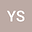loading page

The Brezis-Nirenberg problem for fractional systems with Hardy potentials
•## Abstract

In this work we study the existence of positive solutions to the following fractional elliptic systems with Hardy-type singular potentials, and coupled by critical homogeneous nonlinearities \begin{equation*} \begin{cases} (-\Delta)^{s}u-\mu_{1}\frac{u}{|x|^{2s}}=|u|^{2^{\ast}_{s}-2}u+\frac{\eta\alpha}{2^{\ast}_{s}}|u|^{\alpha-2} |v|^{\beta}u+\frac{1}{2}Q_{u}(u,v) \ \ in \ \Omega, \\[2mm] (-\Delta)^{s}v-\mu_{2}\frac{v}{|x|^{2s}}=|v|^{2^{\ast}_{s}-2}v+\frac{\eta\beta}{2^{\ast}_{s}}|u|^{\alpha} |v|^{\beta-2}v+\frac{1}{2}Q_{v}(u,v) \ \ in \ \Omega, \\[2mm] \ \ u, \ v>0 \ \ \ \ \ in \ \ \Omega, \\[2mm] \ u=v=0 \ \ \ \ in \ \ \mathbb{R}^{N}\backslash\Omega, \end{cases} \end{equation*} where $(-\Delta)^{s}$ denotes the fractional Laplace operator, $\Omega\subset\mathbb{R}^{N}$ is a smooth bounded domain such that $0\in\Omega$, $\mu_{1}, \mu_{2}\in [0,\Lambda_{N,s})$, $\Lambda_{N,s}=2^{2s}\frac{\Gamma^{2}(\frac{N+2s}{4})}{\Gamma^{2}(\frac{N-2s}{4})}$ is the best constant of the fractional Hardy inequality and $2^{*}_{s}=\frac{2N}{N-2s}$ is the fractional critical Sobolev exponent. In order to prove the main result, we establish some refined estimates on the extremal functions of the fractional Hardy-Sobolev type inequalities and we get the existence of positive solutions to the systems through variational methods.

#### Peer review status:UNDER REVIEW

26 Jan 2021Submitted to Mathematical Methods in the Applied Sciences
26 Jan 2021Assigned to Editor
26 Jan 2021Submission Checks Completed
04 Mar 2021Reviewer(s) Assigned
16 Jun 2021Review(s) Completed, Editorial Evaluation Pending